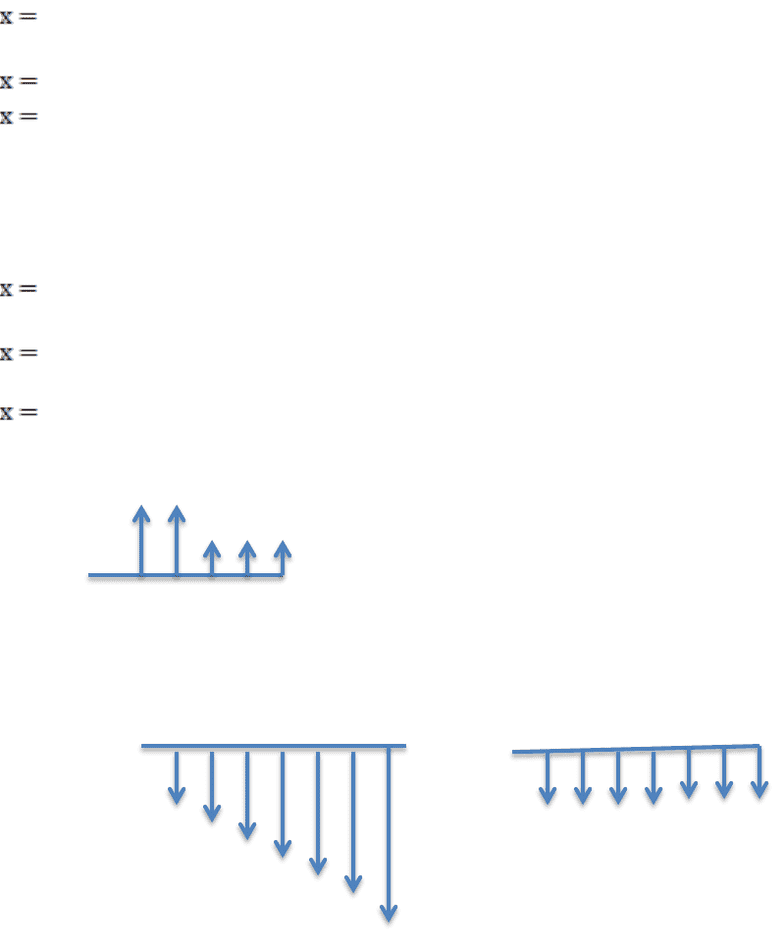Class Notes (1,100,000)
CA (620,000)
UTSG (50,000)
CHE (300)
Lecture 7

# CHE249H1 Lecture Notes - Lecture 7: Interest Rate

Department
Chemical Engineering and Applied Chemistry
Course Code
CHE249H1
Professor
Yuri Lawryshyn
Lecture
7

This preview shows page 1. to view the full 4 pages of the document.CHE374-2015F-Problem Set #6-Solution
Question #1:
Interest rate = 6%
a) Present worth = -\$800 + \$320(P/A,6%,6) = -\$800 +\$320(4.91732)
=\$773.54
b) Future worth = \$773.54 (F/P,6%,6) = \$773.54* 1.4185 = \$ 1097.29
c) \$773.54* (1.06)4= \$976.58
d) \$773.54= x/(1.06) + 5x/(1.06)3 x =\$150.45
Interest rate = 9%
a) Present worth = -\$800 + \$320(P/A,9%,6) = -\$800 +\$320(4.48592)
=\$635.49
b) Future worth =\$635.49 (F/P,9%,6) = \$635.49* 1.6771 = \$ 1065.79
c) \$635.49* (1.09)4= \$ 897.05
d) \$635.49= x/(1.09) + 5x/(1.09)3 x =\$132.99
Question #2: 15K 7K
Value = 15000 (P/A, 8%, 2) +7000(P/A, 8%, 3) (P/F, 8%, 2) = \$50,710.71
Question #3:
=
A = 6K+0.75K(A/G,9%,7)= 6+0.75(2.65740)=\$ 7,993.05
?
6K
∆= 0.75𝐾/𝑦𝑟
###### You're Reading a Preview

Unlock to view full version